# Tutorial: Error Estimation - Part 1 (Introduction and Binning Analysis)¶

## Data generation¶

In this tutorial, you will learn how to estimate the accuracy of your simulation results. Because we are going to emply statistical methods, we need a fair amount of data to play with. The following code cell will generate two data sets which will be used throughout the tutorial.

In :
import numpy as np
%matplotlib inline
import matplotlib.pyplot as plt

In :
plt.rcParams.update({'font.size': 18})
import sys
import logging
logging.basicConfig(level=logging.INFO, stream=sys.stdout)

np.random.seed(43)

def ar_1_process(n_samples, c, phi, eps):
'''
Generate a correlated random sequence with the AR(1) process.

Parameters
----------
n_samples: :obj:int
Sample size.
c: :obj:float
Constant term.
phi: :obj:float
Correlation magnitude.
eps: :obj:float
Shock magnitude.
'''
ys = np.zeros(n_samples)
if abs(phi) >= 1:
raise ValueError("abs(phi) must be smaller than 1.")
# draw initial value from normal distribution with known mean and variance
ys = np.random.normal(loc=c / (1 - phi), scale=np.sqrt(eps**2 / (1 - phi**2)))
for i in range(1, n_samples):
ys[i] = c + phi * ys[i - 1] + np.random.normal(loc=0., scale=eps)
return ys

# generate simulation data using the AR(1) process

logging.info("Generating data sets for the tutorial ...")

N_SAMPLES = 100000

C_1 = 2.0
PHI_1 = 0.85
EPS_1 = 2.0
time_series_1 = ar_1_process(N_SAMPLES, C_1, PHI_1, EPS_1)

C_2 = 0.05
PHI_2 = 0.999
EPS_2 = 1.0
time_series_2 = ar_1_process(N_SAMPLES, C_2, PHI_2, EPS_2)

logging.info("Done")

INFO:root:Generating data sets for the tutorial ...
INFO:root:Done

In :
fig = plt.figure(figsize=(10, 6))
plt.title("The first 1000 samples of both time series")
plt.plot(time_series_1[0:1000], label="time series 1")
plt.plot(time_series_2[0:1000], label="time series 2")
plt.xlabel("$i$")
plt.ylabel("$X_i$")
plt.legend()
plt.show()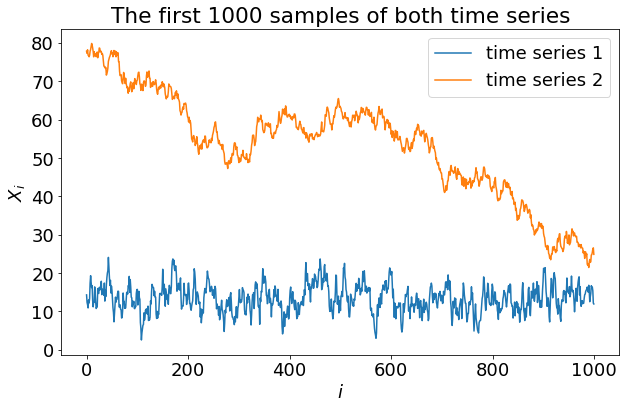## Introduction¶

In this tutorial, you will learn how to do statistical analysis of your simulation data. This is an important topic, because the statistics of your data determine how precise your simulation result is. Furthermore, knowing about the statistics can help you optimize your disk space usage.

ESPResSo provides a lot of ways to take measurements of your system. Usually, you will sample a quantity many times during a simulation and in the end average over all samples. Intuitively, the simulation result will be more precise the more samples are taken during the simulation. However, this is not the whole truth. There are some things that need to be considered, which we will cover in this tutorial.

Formally, if you determine a physical quantity by averaging over several samples, you only approximate the unknown, true mean value. Usually, the quantity is expected to fluctuate around its mean; therefore, you can never directly measure the mean. You are bound to take repeated measurements and in the end average over all samples (a finite number). In your report, you will present this average as your result. Additionally, you should express the precision of your measurements to give a proper meaning to your result. And this is where things get more involved.

There are several different ways to express the precision of your measurements. We will begin by briefly discussing what they are and what their differences are. After that, we will continue with the standard error of the mean as a viable option to be presented in your simulation results.

### Standard deviation¶

The standard deviation is a measure for how much individual samples are expected to deviate from the mean. We want to use precise terminology, and therefore need to state that, in fact, we cannot directly measure the standard deviation but only estimate it. A commonly used estimator for the standard deviation is

\begin{align} \hat{\sigma} = \sqrt{\frac{1}{N-1.5}\sum_{i=1}^{N}(X_i-\overline{X})^2}\tag{1} \end{align}

where $\hat{\sigma}$ is the estimator of the standard deviation $\sigma$, $N$ the number of samples, $X_i$ the individual samples and $\overline{X}$ their mean. This estimator somewhat resembles the "square root of the variance". The curious $-1.5$ in the denominator is a necessary correction to make the estimator less biased (for further reading, see ).

### Standard error of the mean¶

The standard error of the mean (often abbreviated as SEM, or $s$, and its estimator is designated $\hat{\sigma}_\overline{X}$) describes how much the mean value of your sample is expected to deviate from the true mean value $\mu$. Imagine repeating the whole simulation over and over again, taking $N$ samples every time and averaging over them. The SEM quantifies how much those averages will fluctuate around the true mean $\mu$. In fact, it is defined as the standard deviation of the averages.

At first glance, it might seem to be very expensive to compute the SEM, because one would have to repeat the whole simulation many times. However, under the right circumstances, the SEM can be estimated from a single series of $N$ measurements. We will discuss how this can be done.

### Confidence interval¶

A confidence interval (CI) specifies a range of numbers within which the unknown true mean value $\mu$ lies with a certain probability $1-\alpha$. A common confidence level is $1-\alpha=95~\%$. A $95~\%$ CI would contain the true value $\mu$ with probability $95~\%$. Care must be taken interpreting the CI, since the lower and upper bound of a CI are themselves random variables. Just as a simulation run drafts samples from the overall ensemble, determining a CI from a simulation run is drafting a CI from all possible CIs. When the upper and lower bound of a CI have been calculated, this range either contains the true value or not, so there no longer is a probability attached to it. However, for repeated simulations with subsequent computation of the corresponding CIs, on average $95~\%$ of CIs will contain the true value, while $5~\%$ won't.

If the samples are normally distributed and the SEM is known, the upper and lower bounds of the $95~\%$ CI are $\overline{X} \pm 1.96 \, \hat{\sigma}_\overline{X}$.

### Interquartile range¶

The interquartile range denotes the range, within which the central $50~\%$ of all samples lie, if one were to order them by their size. This leaves one quarter of all samples lying below the interquartile range, and another quarter of all samples above it.

### Now – what do we use?

We are interested in the precision of our overall, averaged, simulation result, and not in the precision of the individual samples. Those are expected to fluctuate, and in many cases, those fluctuations are uninteresting for the end result. Out of the options presented above, the SEM and the CI are the only ones doing this requirement justice. Since they are related, the question boils down to how to compute the SEM, which will be the topic of the rest of this tutorial.

## Uncorrelated samples¶

How the SEM can be computed depends on the data itself. For uncorrelated samples, it is nearly trivial:

\begin{align} \hat\sigma_\overline{X} = \frac{\hat\sigma}{\sqrt{N}}\tag{2} \end{align}

where $\hat\sigma_\overline{X}$ is the estimated SEM, $\hat\sigma$ is the estimated standard deviation (see eq. 1) and $N$ is the number of samples. But what does it mean for samples to be uncorrelated?

An example for uncorrelated samples would be the rolling of a dice. The outcome of each trial is completely independent to the previous trials. We might guess any number from 1 to 6, regardless of what has been the last result. The same could be true if we ran an experiment many times independently from one another and measured a quantity each time. By looking at one experimental value, we would'nt be able to predict the next one. The best guess would be simply the mean value of the entire series. In the case of rolling a dice, correlations could for example be observed if it was more probable to obtain the same result as in the previous dice roll rather than another result.

Usually, when you run a molecular dynamics simulation, the particles will only move by a tiny amount during a time step. Consequently, most observables also change only by a small amount during a time step and it is, therefore, more probable to obtain a similar result rather than a completely different result. If we were to sample an observable in every time step, we would get a lot of samples with very similar values. It is said that the samples are correlated. Only if we wait for a sufficiently long time, the system will eventually have evolved to a completely different configuration, and we can expect the observable to assume a truly independent, uncorrelated value.

It is often easy to see when samples are correlated. Execute the code cell below for an example, where a small part of time_series_1 is plotted.

In :
fig = plt.figure(figsize=(10, 6))
plt.plot(time_series_1[1000:1050], "x")
fig.axes.margins(y=0.1)
plt.xlabel("$i$")
plt.ylabel("$X_i$")
plt.show()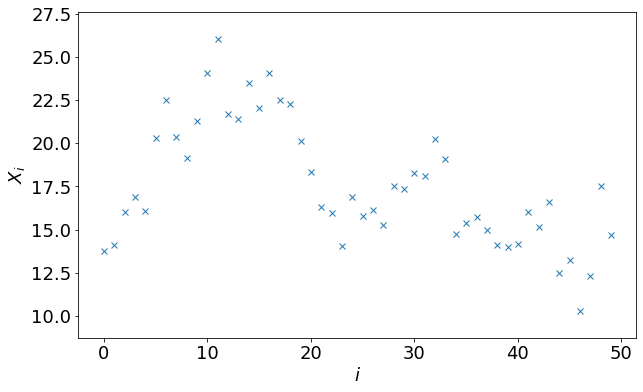One can clearly see that each sample lies in the vicinity of the previous one.

Below is an example for almost completely uncorrelated samples. The data points are taken from the same time series as in the previous example, but this time they are chosen with large gaps in between (every 800th sample is used). These samples appear to fluctuate a lot more randomly.

In :
fig = plt.figure(figsize=(10, 6))
plt.plot(np.arange(2000, 42000, 800), time_series_1[2000:42000:800], "x")
fig.axes.margins(y=0.1)
plt.xlabel("$i$")
plt.ylabel("$X_i$")
fig.axes.xaxis.set_major_locator(plt.MultipleLocator(base=8000))
plt.show()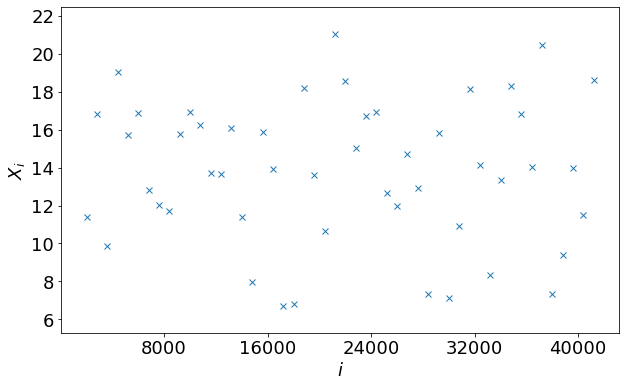However, you should not trust your eye in deciding whether or not a time series is correlated. In fact, when running molecular dynamics simulations, your best guess is to always assume that samples are correlated, and that you should use one of the following techniques for statistical analysis, and rather not just use equation (2).

## Binning analysis¶

Binning analysis is a straightforward method to calculate the SEM for correlated data. A time series of measurements of $N$ samples is divided into $N_\mathrm{B}$ equally long blocks called bins. If $N$ is not an integer multiple of $N_\mathrm{B}$, some data must be discarded to achieve this. The samples in every bin are averaged, giving the bin averages $\overline{X}_i$. It is important that the bin size $N/N_\mathrm{B}$ is significantly larger than the correlation time. Otherwise, binning analysis will yield the wrong SEM.

Once we have computed the bin averages $\overline{X}_i$, getting the SEM is straightforward: we can simply treat $\overline{X}_i$ as an uncorrelated time series. In other words, we can compute the SEM by using equation (1) and (2)!

Let's implement this.

In :
BIN_SIZE = 2000


#### Exercise¶

• Determine the maximally possible number of bins of size BIN_SIZE with the data in time_series_1, and store it in a variable N_BINS.
• Create a numpy array called bin_avgs of length N_BINS.
• Compute the bin averages of time_series_1 and store them in bin_avgs.
In :
N_BINS = N_SAMPLES // BIN_SIZE
bin_avgs = np.zeros(N_BINS)
for i in range(N_BINS):
bin_avgs[i] = np.average(time_series_1[i * BIN_SIZE:(i + 1) * BIN_SIZE])


#### Exercise¶

Compute the average of all bin averages and store it in avg. This is the overall average, our best guess for the measured quantity. Furthermore, compute the standard error of the mean using equations (1) and (2) from the values in bin_avgs and store it in sem.

In :
avg = np.average(bin_avgs)
sem = np.sqrt(np.sum((bin_avgs - avg)**2) / (N_BINS - 1.5) / N_BINS)

In :
print(f"Best guess for measured quantity: {avg:.3f}")
print(f"Standard error of the mean: {sem:.3f}")

Best guess for measured quantity: 13.362
Standard error of the mean: 0.042


Now we already have an estimate on how precise our simulation result is. But how do we know if we chose the appropriate bin size? The answer is, we can perform binning analysis for many different bin sizes and check when the SEM converges. For that we would like to define a function that does the binning analysis in one go.

#### Exercise¶

Define a function called do_binning_analysis that takes as arguments data (a numpy array containing the samples) and bin_size and returns the estimated SEM. You can reuse your code from the previous exercises and adapt it to be part of the function.

In :
def do_binning_analysis(data, bin_size):
n_samples = len(data)
n_bins = n_samples // bin_size
bin_avgs = np.mean(data[:n_bins * bin_size].reshape((n_bins, -1)), axis=1)
return np.std(bin_avgs, ddof=1.5) / np.sqrt(n_bins)


#### Exercise¶

Now take the data in time_series_1 and perform binning analysis for bin sizes from 3 up to 5000 and plot the estimated SEMs against the bin size with logarithmic x axis. Your SEM estimates should be stored in a numpy array called sems.

In :
sizes = np.arange(3, 5001, dtype=int)
sems = np.zeros(5001 - 3, dtype=float)
for s in range(len(sizes)):
sems[s] = do_binning_analysis(time_series_1, sizes[s])

plt.figure(figsize=(10, 6))
plt.plot(sizes, sems, "x")
plt.xscale("log")
plt.xlabel("$N_B$")
plt.ylabel("SEM")
plt.show()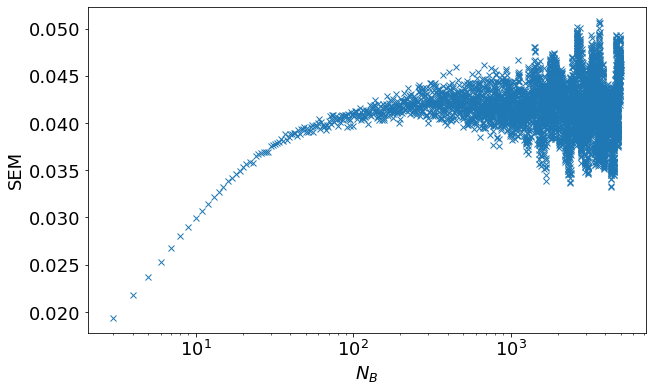You should see that the series converges to a value between 0.04 and 0.05, before transitioning into a noisy tail. The tail becomes increasingly noisy, because as the block size increases, the number of blocks decreases, thus resulting in worse statistics.

To extract the correct SEM from this plot, we can fit an exponential function to the first part of the data, that doesn't suffer from too much noise.

In :
from scipy.optimize import curve_fit

# only fit to the first couple of SEMs
CUTOFF = 600

# sizes of the corresponding bins
sizes_subset = np.arange(3, 3 + CUTOFF, dtype=int)

def fit_fn(x, a, b, c):
return -np.exp(-a * x) * b + c

fit_params, _ = curve_fit(fit_fn, sizes_subset, sems[:CUTOFF], (0.05, 1, 0.5))

fit_sems = fit_fn(sizes, *fit_params)

# compute analytical solutions for AR(1) process
AN_SIGMA_1 = np.sqrt(EPS_1 ** 2 / (1 - PHI_1 ** 2))
AN_TAU_EXP_1 = -1 / np.log(PHI_1)
AN_SEM_1 = np.sqrt(2 * AN_SIGMA_1 ** 2 * AN_TAU_EXP_1 / N_SAMPLES)

plt.figure(figsize=(10, 6))
plt.plot(sizes, sems, "x", label="binning analysis")
plt.plot(sizes[(0, -1),], np.repeat(AN_SEM_1, 2), "-.", label="analytical solution")
plt.plot(sizes, fit_sems, "-", label="fit")
plt.xscale("log")
plt.xlabel("$N_B$")
plt.ylabel("SEM")
plt.legend()
plt.show()

print(f"Final Standard Error of the Mean: {fit_params:.4f}")
print(f"Analytical Standard Error of the Mean: {AN_SEM_1:.4f}")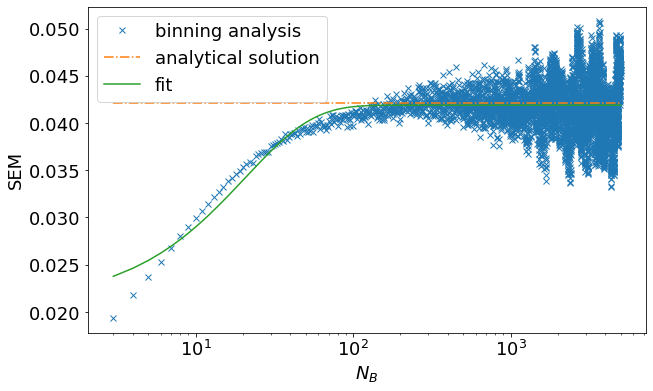Final Standard Error of the Mean: 0.0419
Analytical Standard Error of the Mean: 0.0421


Even though the fit is not perfect, it suffices to give us the position of the asymptote, which is the final estimate for the standard error of the mean. You can see that binning analysis, in fact, managed to estimate the SEM very precisely compared to the analytical solution. This illustrates that most of the time, binning analysis will give you a very reasonable estimate for the SEM, and in fact, is often used in practice because of its simplicity.

However, in some cases, the statistics of your system can be quite challenging. Remember that in real applications, there won't be an analytical solution for the SEM. Therefore, you need to rely entirely on the statistical analysis. It is important to view the statistical analysis critically to decide whether the statistical analysis is trustworthy or not. To illustrate this, let's have a look at the binning analysis of the other time series that was generated at the start of the tutorial:

In :
sizes = np.arange(3, 5001, dtype=int)
sems = np.zeros(5001 - 3, dtype=float)
for s in range(len(sizes)):
sems[s] = do_binning_analysis(time_series_2, sizes[s])

# compute analytical solutions for AR(1) process
AN_SIGMA_2 = np.sqrt(EPS_2 ** 2 / (1 - PHI_2 ** 2))
AN_TAU_EXP_2 = -1 / np.log(PHI_2)
AN_SEM_2 = np.sqrt(2 * AN_SIGMA_2 ** 2 * AN_TAU_EXP_2 / N_SAMPLES)

plt.figure(figsize=(10, 6))
plt.plot(sizes, sems, "x", label="binning analysis")
plt.plot(sizes[(0, -1),], np.repeat(AN_SEM_2, 2), "-.", label="analytical solution")
plt.xscale("log")
plt.xlabel("$N_B$")
plt.ylabel("SEM")
plt.show()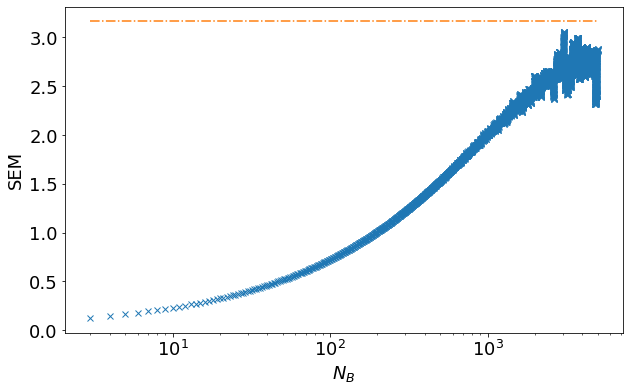Even though we have the exact same number of samples, we cannot see the binning analysis converge. The SEM simply cannot be determined. Usually, this is due to very long correlations, and can only be compensated by simulating for a longer time.

You may notice that the binning analysis gets handwavey and uncertain when the statistics are bad. We could still – despite the noise – fit a function to the above data points and come up with a value for the SEM, but it will most certainly be quite inaccurate and cannot be trusted. For such difficult cases, there is a more rigorous approach to do statistical analysis: Auto-covariance analysis can reveal whether or not your data has sufficient statistics to determine the SEM. It will be discussed in the second part of this tutorial.